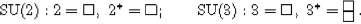Topics, Y

Yamabe Flow > see ADM mass.

Yamabe Invariant
@ References: Botvinnik & Rosenberg JDG(02) [non-simply-connected manifolds]; Avila & Dain CQG(08) [axisymmetric initial data of two Kerr black holes].

Yamabe Question
@ Solution: Schoen JDG(84).

Yang-Baxter Equation > s.a. integrable systems; lie algebras.
* Idea: A relationship between elementary braids in the braid group with n strands.
* Remark: The solutions of the YB equation "at roots of unity" are related to algebraic curves of genus g > 1; They are also known to be connected with the "chiral Potts model".
@ General references: Korepanov in(86)nl/00; Jimbo IJMPA(89) [intro]; Babelon & Viallet pr(89); Perk & Au-Yang en(06)mp [rev]; Nichita a1506 [computational methods and applications].
@ Generalizations: Ambjørn et al NPB(06) [3D, Zamolodchikov's tetrahedron equations, simplified].

Yang-Lee Theory > see under Lee-Yang Theorem / Theory.

Yang-Mills Algebra
@ References: Herscovich & Solotar AM(11) [representations].

Yang-Mills Theories

Yano Tensor
@ References: Cariglia CQG(04)ht/03 [and the Dirac equation in curved spacetime].

Yarkovsky Effect > s.a. Asteroids.
* Idea: A non-gravitational force acting on celestial bodies, e.g., asteroids, from recoil due to re-emission of heat absorbed from the Sun.
@ References: Lorenz & Spitale Icarus(04) [as heat engine]; news BBC(07)mar, pw(07)mar [observation]; De Vos & Kaplar AJP(10)jun [influence on the energy balance and surface temperature]; Vokrouhlický et al a1502-ch [and its rotational variant, the YORP effect].

Yilmaz Theory
* Idea: An alternative, 4D, curved spacetime theory of gravity, in which the Ricci curvature is defined in terms of a massless Klein-Gordon scalar field on an initially flat spacetime, and satisfies the Einstein equation with the usual scalar field stress-energy tensor; It predicts the existence of N-body interacting solutions without event horizons.
@ General references: Yilmaz PR(58), comment Hoffmann JMM(60); Misner NCB(99)gq/95; Alley & Yilmaz NCB(99)gq/95; Wyss NCB(99); Alley et al ap/99/ApJL; Ibison CQG(06)-a0705 [FLRW models].
@ Exponential metric: Martinis & Perković a1009/FP [and Newtonian gravity].

York Time > see time in gravity.

Young Diagrams / Tableaux > s.a. group representations.
* Idea: A diagrammatic way of describing representations of finite and compact Lie groups.
* Examples: For SU(n), denoting the fundamental representation by a box $$\square$$ and the conjugate one by a column of n−1 boxes, we get:* Dimension: The dimension of such a representation can be calculated as follows: ...
@ References: Hamermesh 63; Fulton 96 [applications to representation theory and geometry]; Mironov & Morozov a1912 [hook variables, cut-and-join operators and τ-functions].